Home MathLab Write a Matlab Function That Rotate a Matrix by 90 Degrees

# Write a Matlab Function That Rotate a Matrix by 90 Degrees

We have recently learned how to create functions in Matlab and make use of them. Feel free to have a look at it to know how you will fully make use of the following.

In this session, we are going to see how we can write few lines of code to ask Matlab to help rotate a Matrix for us.

## What do I mean by rotating a Matrix by 90 degrees?

Let’s consider the following Matrix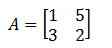What we want to do is building a second matrix based on the matrix A, which is going look like this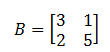B is A rotated by 90 degrees.

In other words what we are trying to accomplish is the following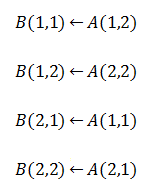## Rotate a Matrix

The code

```N=length(A);
for i=1:N
for j=1:N
B(j,N-i+1)=A(i,j);
end
end```

Here is the code you can use and test on a matrix of your choice. In order to create a function that will handle just this task, you can use this code appropriately.

## Turn columns into rows and vice versa

Turning rows into columns and columns into rows while operating with matrices is called determining the transpose of an already known matrix. If we have a Matrix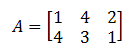and we want to convert rows into columns for it to look like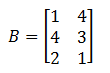We simply need to use the following code in Matlab

`B=A'`

So we will say that A is a transpose of B.

Here is an example of a 3 X 3 matrix and its transpose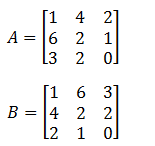There are many already integrated functions in Matlab allowing you to gently play with Matrices. Here are some Matlab Matrix operations you can make use of to make your life easier.